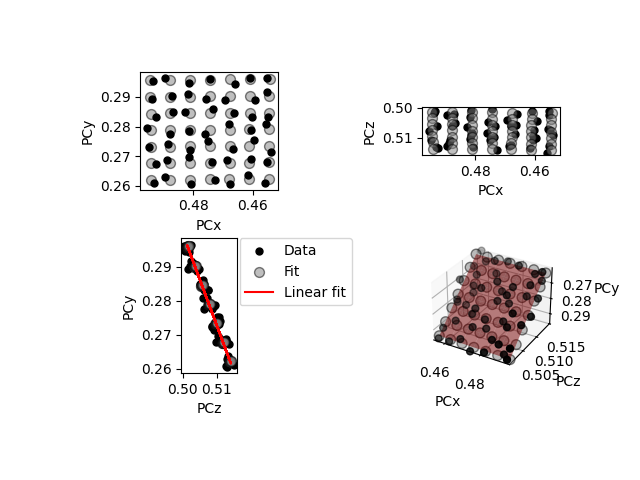# Fit a plane to selected projection centers#

This example shows how to fit a plane to selected projection centers (PCs) using a projective transformation, following .

To test the fit, we add some noise to realistic projection center (PC) values (PCx, PCy, PCz) and fit a plane to a few of the PCs. The realistic PCs are extrapolated from a PC in the upper left corner of a map, assuming a nominal sample tilt of 70 degrees, a detector tilt of 0 degrees, a detector pixel size of 70 microns and a sample step size of 50 microns.```Max error in (PCx, PCy, PCz): [0.00290747 0.0033027  0.00279131]
Estimated sample tilt [deg]: 69.24
```

```import kikuchipy as kp
import matplotlib.pyplot as plt
import numpy as np

plt.rcParams["font.size"] = 10

# Create an initial detector with one PC assumed to be for the upper
# left corner of a map
det0 = kp.detectors.EBSDDetector(
shape=(480, 640),
pc=(0.5, 0.3, 0.5),
sample_tilt=70,
tilt=0,
px_size=70,
)

# Extrapolate a map of PCs
nav_shape = (30, 45)
det1 = det0.extrapolate_pc(
pc_indices=[0, 0],
step_sizes=(50, 50),
)

rng = np.random.default_rng()
dev = 0.002

# Extract a (7, 7) grid of PCs
grid_shape = (7, 7)
pc_indices = kp.signals.util.grid_indices(grid_shape, nav_shape=nav_shape)
det2 = det1.deepcopy()
det2.pc = det2.pc[tuple(pc_indices)].reshape(grid_shape + (3,))

# Get a plane of PCs and plot the match at the same time
map_indices = np.stack(np.indices(nav_shape))
det_fit = det2.fit_pc(pc_indices=pc_indices, map_indices=map_indices)

# Inspect the max. error and sample tilt
max_err = abs(det_fit.pc_flattened - det1.pc_flattened).max(axis=0)
print("Max error in (PCx, PCy, PCz):", max_err)
print(f"Estimated sample tilt [deg]: {det_fit.sample_tilt:.2f}")
```

Total running time of the script: ( 0 minutes 0.832 seconds)

Estimated memory usage: 11 MB

Gallery generated by Sphinx-Gallery